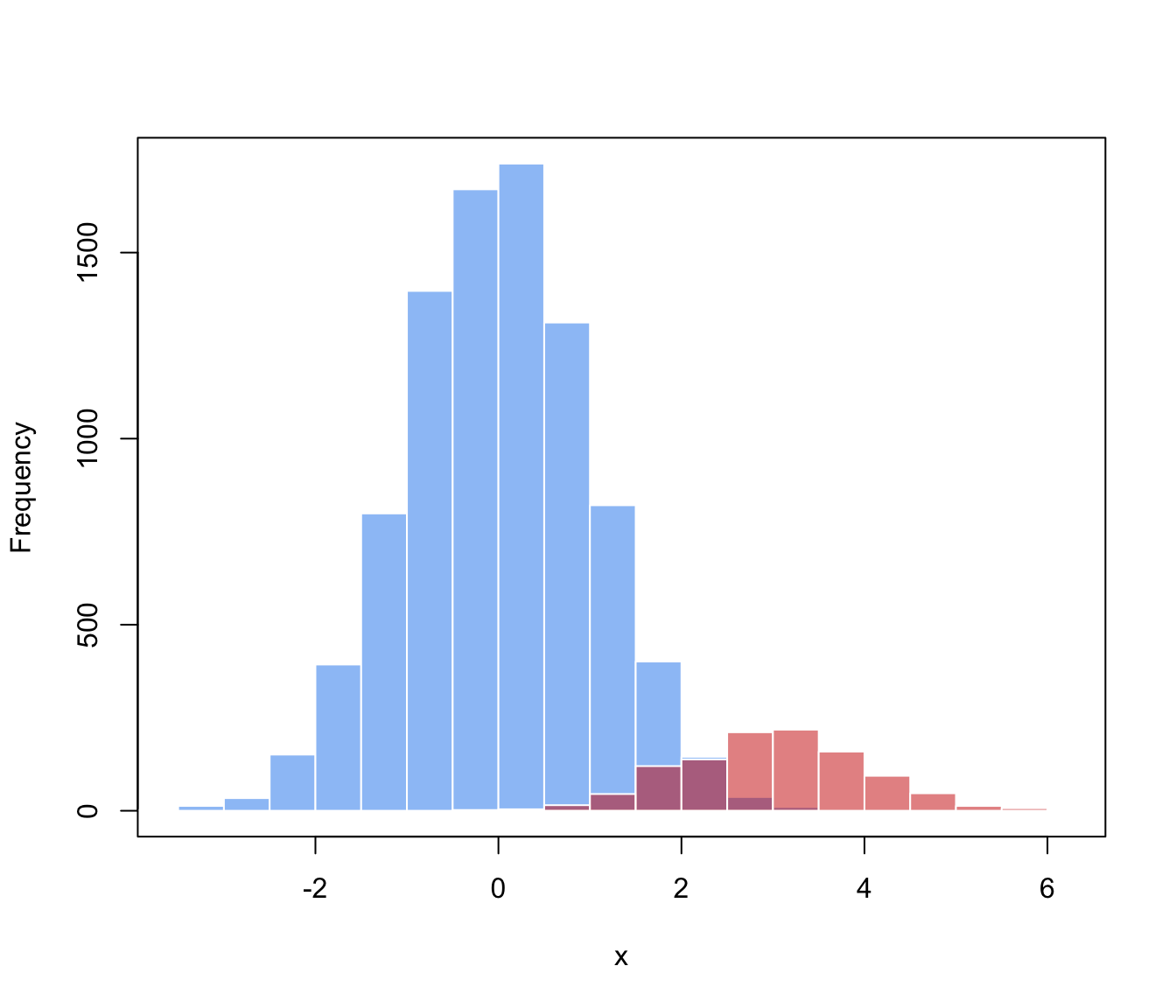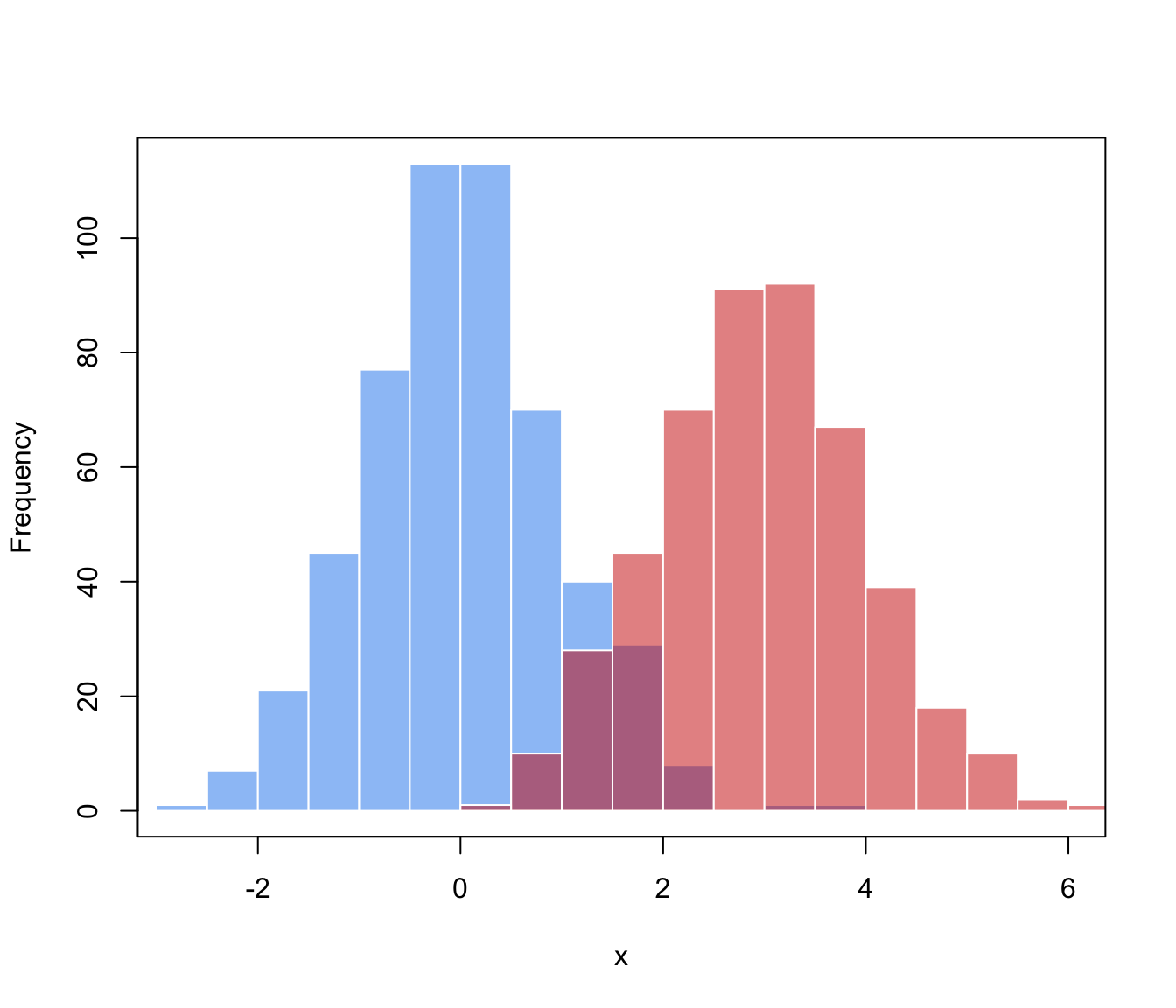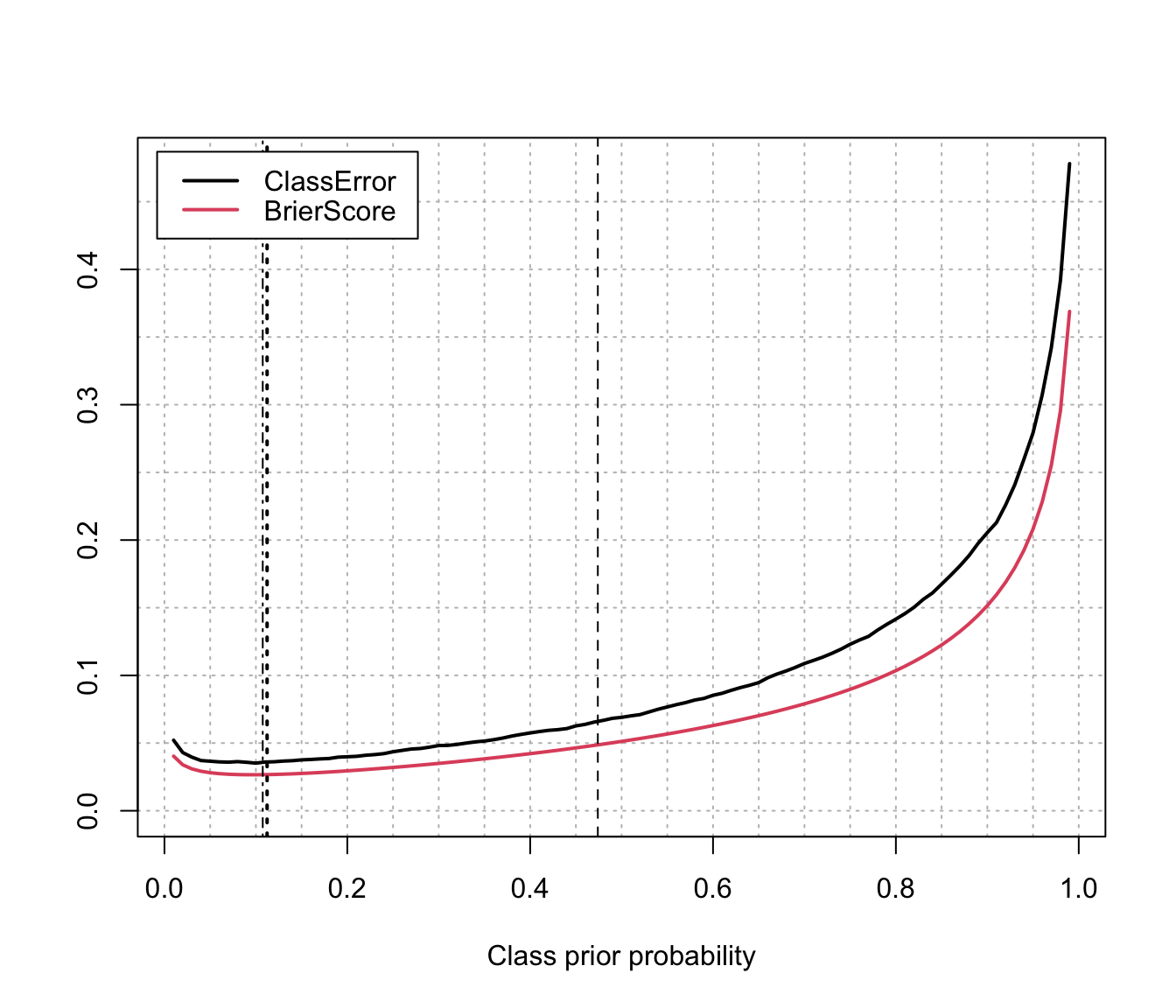A simple procedure to improve the estimation of class prior probabilities when the training data does not reflect the true a priori probabilities of the target classes. The EM algorithm used is described in Saerens et al (2002).

classPriorProbs(object, newdata = object$data, itmax = 1e3, eps = sqrt(.Machine$double.eps))

Arguments

object

an object of class 'MclustDA' resulting from a call to MclustDA.

newdata

a data frame or matrix giving the data. If missing the train data obtained from the call to MclustDA are used.

itmax

an integer value specifying the maximal number of EM iterations.

eps

a scalar specifying the tolerance associated with deciding when to terminate the EM iterations.

Details

The estimation procedure employes an EM algorithm as described in Saerens et al (2002).

Value

A vector of class prior estimates which can then be used in the predict.MclustDA to improve predictions.

Examples

# \donttest{
# generate data from a mixture f(x) = 0.9 * N(0,1) + 0.1 * N(3,1)
n <- 10000
mixpro <- c(0.9, 0.1)
class <- factor(sample(0:1, size = n, prob = mixpro, replace = TRUE))
x <- ifelse(class == 1, rnorm(n, mean = 3, sd = 1),
rnorm(n, mean = 0, sd = 1))

hist(x[class==0], breaks = 11, xlim = range(x), main = "", xlab = "x",
col = adjustcolor("dodgerblue2", alpha.f = 0.5), border = "white")
hist(x[class==1], breaks = 11, add = TRUE,
col = adjustcolor("red3", alpha.f = 0.5), border = "white")
box()# generate training data from a balanced case-control sample, i.e.
# f(x) = 0.5 * N(0,1) + 0.5 * N(3,1)
n_train <- 1000
class_train <- factor(sample(0:1, size = n_train, prob = c(0.5, 0.5), replace = TRUE))
x_train <- ifelse(class_train == 1, rnorm(n_train, mean = 3, sd = 1),
rnorm(n_train, mean = 0, sd = 1))

hist(x_train[class_train==0], breaks = 11, xlim = range(x_train),
main = "", xlab = "x",
col = adjustcolor("dodgerblue2", alpha.f = 0.5), border = "white")
hist(x_train[class_train==1], breaks = 11, add = TRUE,
col = adjustcolor("red3", alpha.f = 0.5), border = "white")
box()# fit a MclustDA model
mod <- MclustDA(x_train, class_train)
summary(mod, parameters = TRUE)
#> ------------------------------------------------
#> Gaussian finite mixture model for classification
#> ------------------------------------------------
#>
#> MclustDA model summary:
#>
#>  log-likelihood    n df       BIC
#>       -1942.376 1000  4 -3912.383
#>
#> Classes   n    % Model G
#>       0 524 52.4     X 1
#>       1 476 47.6     X 1
#>
#> Class prior probabilities:
#>     0     1
#> 0.524 0.476
#>
#> Class = 0
#>
#> Mixing probabilities: 1
#>
#> Means:
#>  -0.03369754
#>
#> Variances:
#>  0.9532879
#>
#> Class = 1
#>
#> Mixing probabilities: 1
#>
#> Means:
#>  2.955709
#>
#> Variances:
#>  1.059664
#>
#> Training confusion matrix:
#>      Predicted
#> Class   0   1
#>     0 488  36
#>     1  29 447
#> Classification error = 0.065
#> Brier score          = 0.0492

# test set performance
pred <- predict(mod, newdata = x)
classError(pred$classification, class)$error
#>  0.0688
BrierScore(pred$z, class) #>  0.05133817 # compute performance over a grid of prior probs priorProp <- seq(0.01, 0.99, by = 0.01) CE <- BS <- rep(as.double(NA), length(priorProp)) for(i in seq(priorProp)) { pred <- predict(mod, newdata = x, prop = c(1-priorProp[i], priorProp[i])) CE[i] <- classError(pred$classification, class = class)$error BS[i] <- BrierScore(pred$z, class)
}

# estimate the optimal class prior probs
(priorProbs <- classPriorProbs(mod, x))
#>         0         1
#> 0.8852142 0.1147858
pred <- predict(mod, newdata = x, prop = priorProbs)
# compute performance at the estimated class prior probs
classError(pred$classification, class = class)$error
#>  0.0352
BrierScore(pred$z, class) #>  0.02658523 matplot(priorProp, cbind(CE,BS), type = "l", lty = 1, lwd = 2, xlab = "Class prior probability", ylab = "", ylim = c(0,max(CE,BS)), panel.first = { abline(h = seq(0,1,by=0.05), col = "grey", lty = 3) abline(v = seq(0,1,by=0.05), col = "grey", lty = 3) }) abline(v = mod$prop, lty = 2)              # training prop
abline(v = mean(class==1), lty = 4)           # test prop (usually unknown)
abline(v = priorProbs, lty = 3, lwd = 2)      # estimated prior probs
legend("topleft", legend = c("ClassError", "BrierScore"),
col = 1:2, lty = 1, lwd = 2, inset = 0.02)# Summary of results:
priorProp[which.min(CE)] # best prior of class 1 according to classification error
#>  0.11
priorProp[which.min(BS)] # best prior of class 1 according to Brier score
#>  0.09
priorProbs               # optimal estimated class prior probabilities
#>         0         1
#> 0.8852142 0.1147858
# }